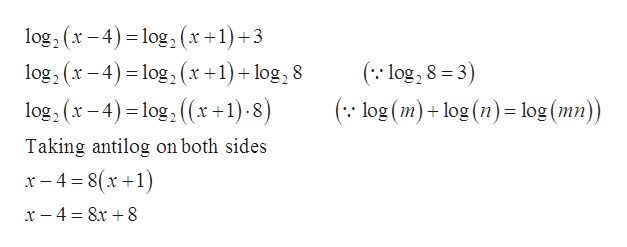Question
26 views

For Exercise, solve the equation.

log2(x − 4) = log2(x + 1) + 3

check_circle

Step 1

To solve the given equation.

Step 2

Given information:

The equation is given below.

Step 3

Calculation:

Solve the given eq...help_outlineImage Transcriptioncloselog, (x – 4) = log, (x +1) +3 log, (x - 4) = log, (x +1) + log, 8 (:: log, 8 = 3) %3D (:: log (m)+ log (n) = log (mn)) log, (x - 4) = log, ((x+1)-8) %3D Taking antilog on both sides x- 4 = 8(x +1) x – 4 = 8x + 8 fullscreen

### Want to see the full answer?

See Solution

#### Want to see this answer and more?

Solutions are written by subject experts who are available 24/7. Questions are typically answered within 1 hour.*

See Solution
*Response times may vary by subject and question.
Tagged in

### Logarithms